# Regression with Gaussian Processes in Clojure

In a previous post I wrote some Clojure code to sample and plot functions from a Gaussian process. That exercise was very helpful for me to learn some of the basics of Gaussian processes, but at the end of the day sampling functions like that doesn’t seem terribly useful, though it does lead to some neat plots. In this post we will take the next step and find a Gaussian process that will generate functions to fit some data. Like my previous post, this will closely follow the examples in chapter 2 of Gaussian Processes for Machine Learning by Rasmussen and Williams, available here. The code from this post is here.

## Prediction with Noiseless Data

We are going to take the GP from the last post

(1)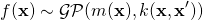as a prior and find a posterior distribution that describes functions characterized by the kernel,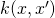, but conditioned on some training data. If we take our set of training inputs,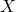, and test inputs,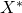, then by the definition of Gaussian processes, the functions values for all of these inputs are jointly Gaussian with covariance given by the kernel,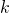.

(2)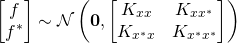I’m using some notation here that I don’t particularly like, so let me try to explain. If we have two sets of pointsand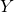,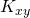is a matrix of values of the covariance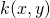evaluated at all pairs of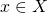and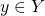.

What we need is a posterior distribution that restricts this GP to functions consistent with the training data. The test predictions,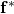, corresponding to inputs,, are found by conditioning the GP on the training:

(3)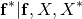Fortunately this is a well-known result we can look up. For jointly Gaussian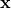and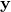(4)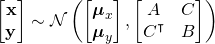we can write

(5)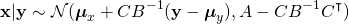or

(6)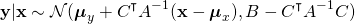which means that the posterior GP is just:

(7)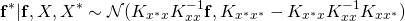The rest is almost embarrassingly simple because we do exactly what we did with the prior GP. We just have a different mean and covariance.

Let’s say we have the following observed values of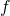at a few points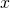(def train-xs [-4 -3 -1 0 1])
(def train-fs [-2 0 1 2 -1])


and we’ll use the same test points, test-xs from the previous post:

This little function computes the common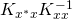term in the new mean and covariance:

(defn k-k-inv* [cov-fn test-xs train-xs]
(let [k* (m/matrix (cov-fn test-xs train-xs))
k (m/matrix (cov-fn train-xs train-xs))]
(m/mmul k* (m/inverse k))))


These functions will compute the new mean and covariance matrix given the test points, training data, and a covariance function.

(defn posterior-mean [cov-fn test-xs train-xs train-fs]
(m/to-nested-vectors
(m/mmul (k-k-inv* cov-fn test-xs train-xs)
(m/matrix train-fs))))
(defn posterior-covariance [cov-fn test-xs train-xs]
(m/to-nested-vectors
(m/sub (m/matrix (cov-fn test-xs test-xs))
(m/mmul (k-k-inv* cov-fn test-xs train-xs)
(m/matrix (cov-fn train-xs test-xs))))))


Now start the plot server up again:

(start-plot-server!)

then compute and plot the results using the squared exponential covariance function

(8)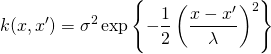defined in sq-exp-cov from the previous post along with the functions line-data, conf-data, and plot-gp.

(let [cov-fn (partial sq-exp-cov 1 1)
post-mean (posterior-mean cov-fn test-xs train-xs train-fs)
post-cov (posterior-covariance cov-fn test-xs train-xs)
post-data (line-data test-xs post-mean post-cov 3 "posterior")
post-conf-data (conf-data test-xs post-mean post-cov)]
(plot-gp post-data post-conf-data))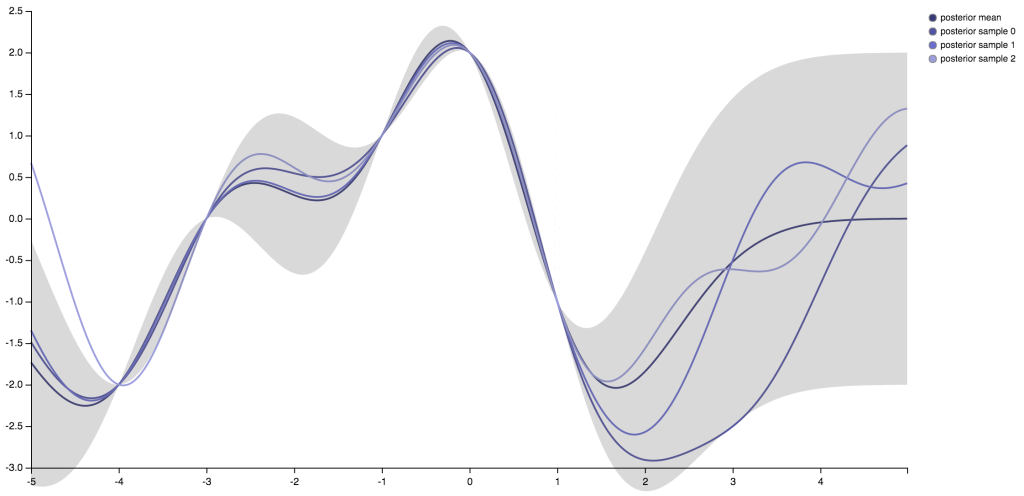Unlike the functions we sampled from the GP in the previous blog post, these functions, including the mean, all pass through the training points. The confidence intervals shrink as the functions approach those points as well. Another interesting thing is that past the last data point on the right, the mean function goes back to zero and the confidence intervals widen out to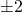again. As we move away from the data, the prior becomes the bigger influence on the properties of.

I don’t know if sampling from a GP is ever useful in practice. The really useful things for prediction are the mean and covariance functions of the the posterior process. Now let’s take a look at how those predictions change when we drop the assumption of noiseless data.

## Prediction with Noise

Where exactly did we assume there was no noise? We actually did this with our choice of covariance function for the training data. If we assume our observations are influenced by some additive i.i.d. Gaussian noise with variance,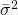, the covariance matrix for those observations goes from being just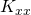to

(9)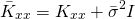Note thatis different from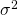which we saw in the covariance function. The magnitude of the noise is defined by, andcontrols the strength of the correlation.

It’s a simple matter to replace theterms in our previous expressions for the mean and covariance of the posterior GP with the version with the noise term.

(10)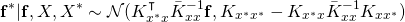And the rest is very similar to what we did before. First we’ll slightly alter the k-k-inv* function to account for noise.

(defn k-k-inv* [cov-fn sigma2-bar test-xs train-xs]
(let [n (count train-xs)
k* (m/matrix (cov-fn test-xs train-xs))
k (m/matrix (cov-fn train-xs train-xs))]
(m/mmul k*
(m/inverse
(m/scale (m/identity-matrix n) sigma2-bar))))))


Now we redefine these functions:

(defn posterior-mean
[cov-fn sigma2-bar test-xs train-xs train-fs]
(m/to-nested-vectors
(m/mmul (k-k-inv* cov-fn sigma2-bar test-xs train-xs)
(m/matrix train-fs))))
(defn posterior-covariance
[cov-fn sigma2-bar test-xs train-xs]
(m/to-nested-vectors
(m/sub (m/matrix (cov-fn test-xs test-xs))
(m/mmul (k-k-inv* cov-fn sigma2-bar test-xs train-xs)
(m/matrix (cov-fn train-xs test-xs))))))


and plot the results

(let [lambda 1.0
sigma2 1.0
sigma2-bar 0.05
cov-fn (partial sq-exp-cov sigma2 lambda)
post-mean (posterior-mean
cov-fn sigma2-bar test-xs train-xs train-fs)
post-cov (posterior-covariance cov-fn sigma2-bar test-xs train-xs)
post-data (line-data test-xs post-mean post-cov 3 "posterior")
post-conf-data (conf-data test-xs post-mean post-cov)]
(plot-gp post-data post-conf-data))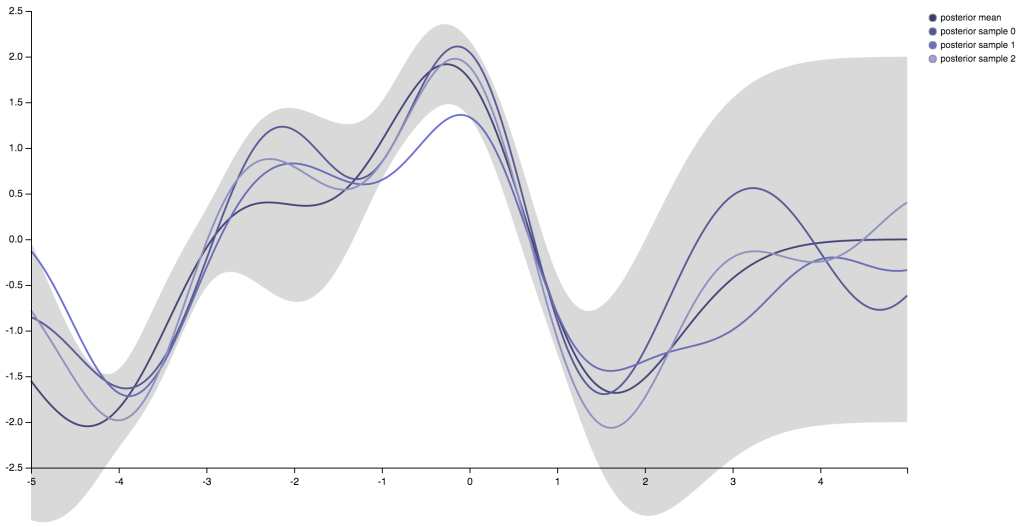This is similar to what we saw before, but now the functions do not pass directly though the training points and the variance does not shrink to zero. Running this code with larger values forwill increase the uncertainty of the observations so the variance at those points will get larger and values of the functions sampled at and near those inputs will more dispersed around the observed values of.

## Playing with Hyperparameters

The squared exponential covariance function has a couple of hyperparameters that we’ve set to 1.0 and ignored for the most part. In a fully Bayesian treatment we define prior distribution for those values. I hope to get to this eventually, but for now I just want to demonstrate what they represent and how they influence the regression results.is fairly easy. Increasing or decreasing it will make the functions described by the Gaussian process more or less likely to vary far away from the mean.

(let [lambda 0.1
sigma2 4.0
sigma2-bar 0.05
cov-fn (partial sq-exp-cov sigma2 lambda)
post-mean (posterior-mean
cov-fn sigma2-bar test-xs train-xs train-fs)
post-cov (posterior-covariance cov-fn sigma2-bar test-xs train-xs)
post-data (line-data
test-xs post-mean post-cov 3 "larger variance")
post-conf-data (conf-data test-xs post-mean post-cov)]
(plot-gp post-data post-conf-data))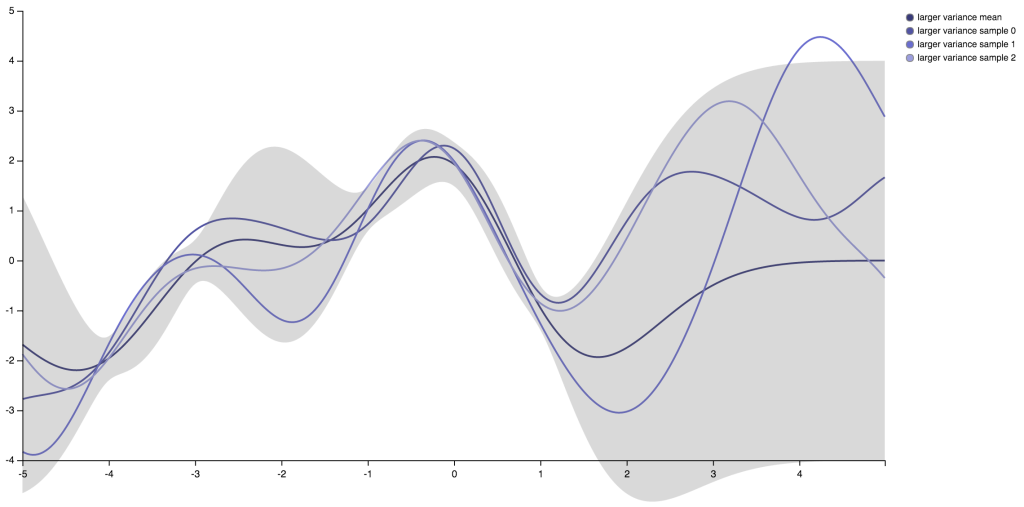The parameter,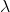, is a little more interesting. It gives us the scale over which points can be considered similar. We don’t expect function to vary much over ranges around the size ofor smaller. So, for example, if we reducewe will see the functions produced from the GP to wiggle around on smaller scales. And if we increase, we can expect those functions to vary less across the range of test points than they do now.

(let [lambda 0.1
sigma2 1.0
sigma2-bar 0.05
cov-fn (partial sq-exp-cov sigma2 lambda)
post-mean (posterior-mean
cov-fn sigma2-bar test-xs train-xs train-fs)
post-cov (posterior-covariance cov-fn sigma2-bar test-xs train-xs)
post-data (line-data
test-xs post-mean post-cov 3 "smaller lambda")
post-conf-data (conf-data test-xs post-mean post-cov)]
(plot-gp post-data post-conf-data))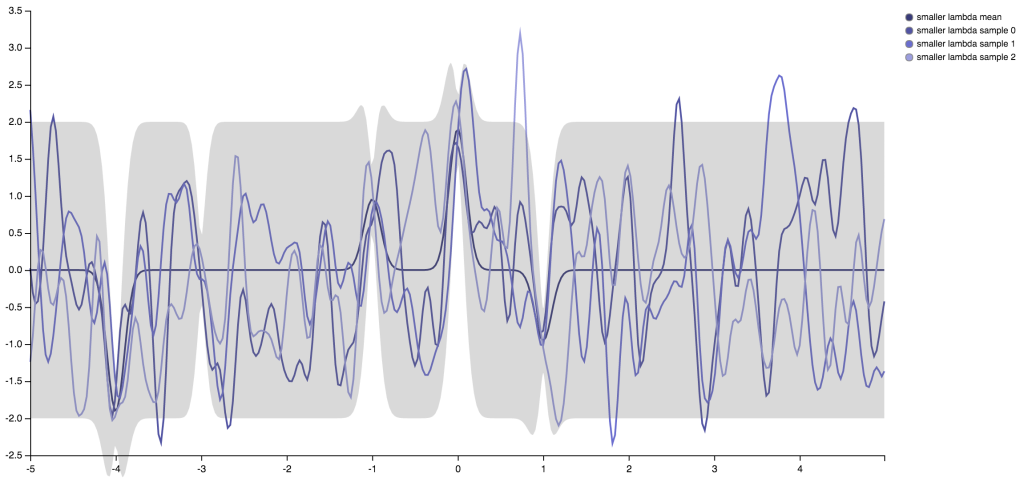(let [lambda 2.0
sigma2 1.0
sigma2-bar 0.05
cov-fn (partial sq-exp-cov sigma2 lambda)
post-mean (posterior-mean
cov-fn sigma2-bar test-xs train-xs train-fs)
post-cov (posterior-covariance cov-fn sigma2-bar test-xs train-xs)
post-data (line-data test-xs post-mean post-cov 3 "larger lambda")
post-conf-data (conf-data test-xs post-mean post-cov)]
(plot-gp post-data post-conf-data))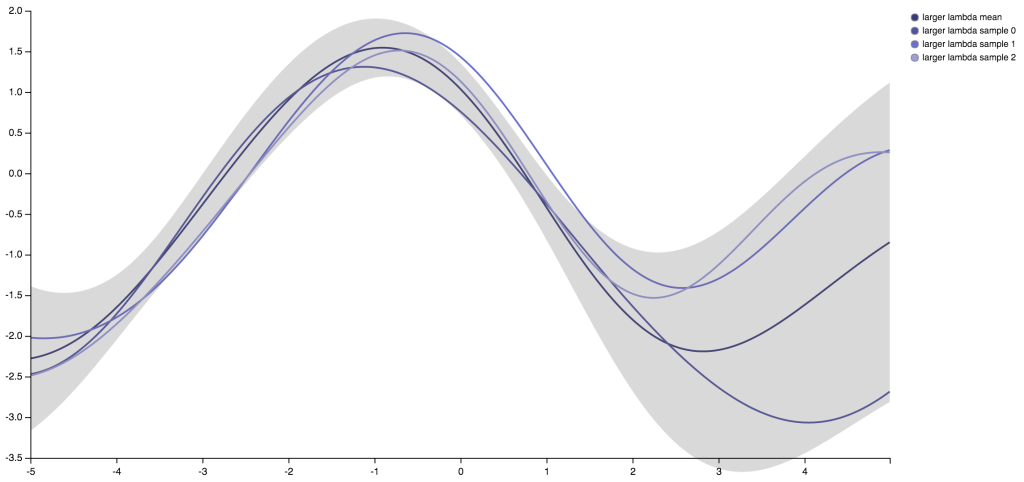## Closing Remarks

The nice thing about everything we’ve seen, aside from it being done entirely within a Clojure REPL, is how simple it is. Once we have a covariance function picked out, the hardest thing we needed to do was invert a matrix. Fortunately, core.matrix made the linear algebra easy. Just a few matrix operations and we have a very flexible way to perform regression.

In future posts, I plan to explore using Gaussian processes for time series forecasting. I will also spend more time looking at alternative covariance functions and address the problem of optimizing the hyperparameters.